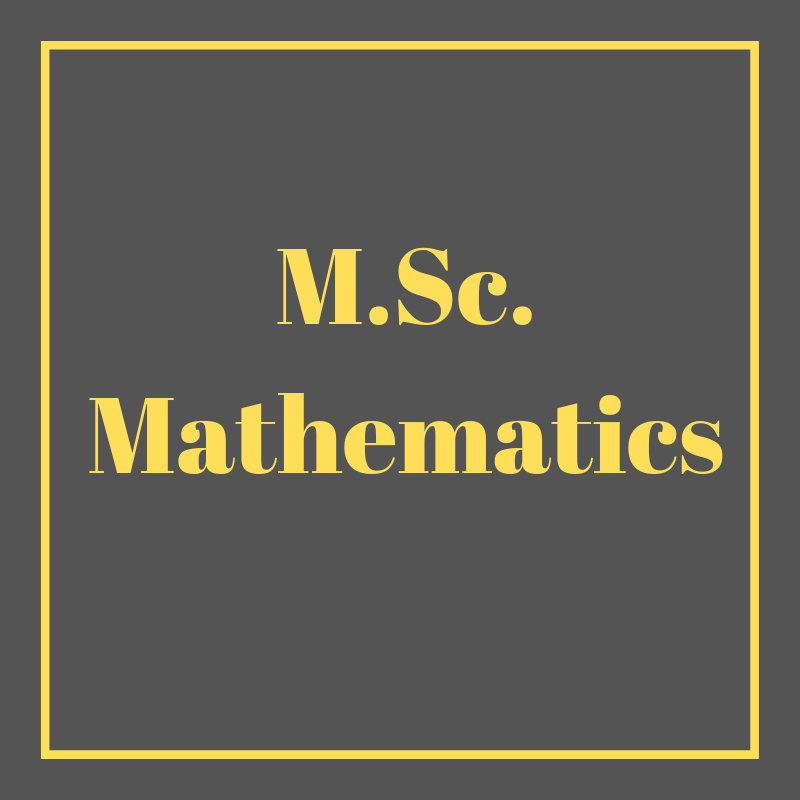# M.Sc. (Mathematics)## Objectives:

#### The course aims to:

• Prepare the students with the essential tools of algebra
• Develop skills to apply the concepts and the techniques

#### Course Contents:

Preliminaries: Real-number system, complex numbers, introduction to sets, set operations, functions, types of functions.
Matrices:  Introduction to matrices, types, matrix inverse, determinants, system of linear equations, Cramer’s rule.
Quadratic Equations: Solution of quadratic equations, qualitative analysis of roots of a quadratic equations, equations reducible to quadratic equations, cube roots of unity, relation between roots and coefficients of quadratic equations.
Sequences and Series:  Arithmetic progression, geometric progression, harmonic progression. Binomial Theorem: Introduction to mathematical induction, binomial theorem with rational and irrational indices. Trigonometry: Fundamentals of trigonometry, trigonometric identities.